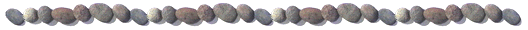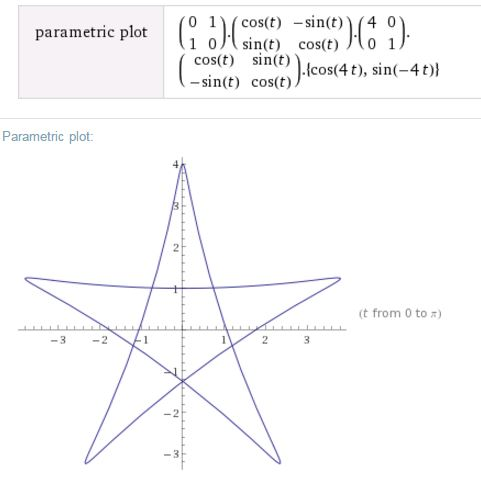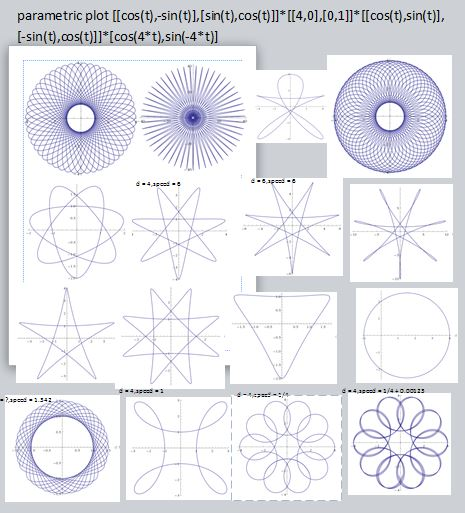### Spiral Graph EquationsI have been playing with rotating an ellipse at speed 1, while moving a point around the ellipse at a different speed s. The dimensions of the ellipse are 1 by d. It gives fun spiral graph pictures.
The pentacle and triangle with almost straight edges surprised me. An n (odd) pointed one is made with d=n-1 and s= -(n-1). But the second row shows s=-6 with more and more skinny ellipses. The first has convex lines and the last concave, so there has to be a place in the middle.
The shifted circle with d=4 and s=2 is also fun.
Of course the bottom right was given thickness by adding an small shift to s.
The 3rd was a mistake.
The trials of not being busy teaching. <3

wolframalpha
parametric plot [[0,1],[1,0]]*[[cos(t),-sin(t)],[sin(t),cos(t)]]*[[4,0],[0,1]]*[[cos(t),sin(t)],[-sin(t),cos(t)]]*[cos(-4*t),sin(-4*t)]
[cos(-4*t),sin(-4*t)] moves the point around a circle at speed s=-4.
[[cos(t),sin(t)],[-sin(t),cos(t)]] rotates this point by an angle of t.
[[4,0],[0,1]] stretches it in the x direction by d=4.
[[cos(t),-sin(t)],[sin(t),cos(t)]] rotates it back the angle of t.
[[0,1],[1,0]] rotate the peak to the top.
"parametric plot" plots it for values of t.